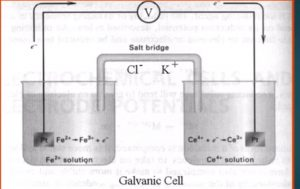# REDOX TITRATIONS: Theory, Concept, Electrochemical Cell and MCQ for GPAT, GATE, and NET JRF exam

## REDOX TITRATIONS: Theory, Concept, Electrochemical Cell and MCQ for GPAT, GATE, and NET JRF exam

### OXIDATION :-

• Oxidation is gain of oxygen
• Oxidation is loss of hydrogen
• Oxidation is loss of electrons

### REDUCTION :-

• Reduction is loss of oxygen.
• Reduction is gain of hydrogen
• Reduction is gain of electrons.

### Rule for Assigning oxidation number :-

1. The oxidation number of any uncombined element is zero
2. The oxidation number of a monatomic ion is equal its change.
3. The oxidation number of oxygen in compound is -2 except in peroxide such as H2O2 where it is -1.
4. The oxidation number of hydrogen in compound is +1 except in metal hybribes like NaH where it is -1.
5. The sum of the oxidation number of the atom in the compound must equal 0.

STANDARD REDUCTION POTENTIAL (SRP) :-

The standard reduction potential is tendency for a chemical species to be reduced and is measured in volts at standard condition.

The standard potential are all measured at 298 K, 1 ATM and 1 M solution.

The more positive the potential is the more likely it will be reduced.

It is designated as Eº.

### STANDARD OXIDATION POTENTIAL (SOP):-

It is the tendency for a species to be oxidized at standard condition.

Eº (SRP) =-Eº(SOP)

### ELECTROCHEMICAL CELLS :-

• A Redox Titrationis based on reaction of oxidation and reduction between analyte and titrant.
• This reaction are studied and measuring by electrochemical cell. In electrochemical cell we are measuring changing in potential of the cell.There are mainly two types of electrochemical cell

1. Galvanic cell
2. Electrolytic cell

1.Galvanic cell :- In this type of cell spontaneously produces chemical reaction and generate electrical energy.

2. Electrolytic cell :-  In this type of cell by using electrical energy we performed chemical reaction.

Galvanic cell are of importance in our further discussion as we will be discussing the spontaneous chemical reaction to produced electrical energy

Consider the titration of iron (II) with standard cerium (IV)

Monitored by potentiometrically.

If a solution containing Fe2 is mixed with another solution containing Ce4 there will be a redox reaction situation due to their tendency of transfer electrons.

If we consider that these two solution are kept in separate beaker and connected by salt bridge and a platinum wire that will become a galvanic cell.

If we connect a voltmeter between two electrode, the potential difference of two electrode can be directly measured.
The Fe2 Is being oxidised at the platinum wire (the anode):-

Fe2  →   Fe+ e

The electron thus produced will flow through the wire to the other beaker where the Ce4 is reduced (at the cathode).

Ce4 + e–     Ce3

Before we start the discussion of the oxidation reduction titration curve construction, we should understand the Nernst equation which was introduced by German scientist, Walter Nernst in 1889.
This equation express the relation between the potential of metal or metal ion and the concentration of the ion in the solution.
Lets consider the following chemical reaction :—
aA +bB     ⇔   cC+dD
The change In free energy is given by the equation

∆G = ∆ Go + 2.3 RT Log [C]c [D]d/[A]a [B]b

After dividing both side with -nF, we can get the expression as

E=E°-2.3RT/ -nf   Log [C]c [D]d/[A]a [B]b

At 25°C, the equation can be written as

E=E° + 0.095/n  Log [C]c [D]d/[A]a [B]b

THIS CAN BE GENERALIZED AS :-

E=E° +0.095\n Log [oxd.]/[Red]

This equation is known as the “Nernst equation” that correlate the electrode potential with the concentration of the ionic species.

### MCQ

1.Which sentence is flase about oxidation ?

a.Loss of electrons

b.Gain of oxygen

c.Loss of oxygen

d. Loss of hydrogen

2. SOP is

a.STANDARD REDUCTION PRESSURE

b.STANDARD REDUCTION POTENTIAL

c.STANDARD OXIDATION PRESSURE

d.STANDARD OXIDATION POTENTIAL

3.SRP is

a.STANDARD REDUCTION PRESSURE

b.STANDARD REDUCTION POTENTIAL

c.STANDARD OXIDATION PRESSURE

d.STANDARD OXIDATION POTENTIAL

4. Which sentence is false about Reduction ?

a.Loss of oxygen.

b. Gain of hydrogen

c. Loss of electron

d.Gain of electron

5.Electrochemical cell is

a. Measuring changing in potential

b.Convert chemical energy to electrical energy

c.Measuring redox reaction

d. Study of internal structure of compound

6. Electrolytic cell is

a.Produces chemical reaction and generate electrical energy

b.By using electrical energy we performed chemical reaction

c. a and b

d.None of the above

7. Galvanic cell is

a.Produces chemical reaction and generate electrical energy

b.By using electrical energy we performed chemical reaction

c. a and b

d.None of the above

1.c

2.d

3.b

4.d

5.d

6.a

7.b